# INDEX & MATCH in Excel - better alternative to VLOOKUP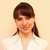by , updated on

This tutorial shows how to use INDEX and MATCH in Excel and how it is better than VLOOKUP.

In a couple of recent articles, we made a good effort to explain the basics of VLOOKUP function to beginners and provide more complex VLOOKUP formula examples to power users. And now, I will try if not to talk you out of using VLOOKUP, then at least show you an alternative way to do a vertical lookup in Excel.

"What do I need that for?" you may wonder. Because VLOOKUP has numerous limitations that can prevent you from getting the desired result in many situations. On the other hand, the INDEX MATCH combination is more flexible and has many awesome features that make it superior to VLOOKUP in many respects.## Excel INDEX and MATCH functions - the basics

Since the aim of this tutorial is to demonstrate an alternative way to do a vlookup in Excel by using a combination of INDEX and MATCH functions, we won't dwell much on their syntax and uses. We will cover only the minimum necessary for understanding the general idea and then take an in-depth look at formula examples that reveal all the advantages of using INDEX MATCH instead of VLOOKUP.

### INDEX function - syntax and usage

The Excel INDEX function returns a value in an array based on the row and column numbers you specify. The syntax of the INDEX function is straightforward:

INDEX(array, row_num, [column_num])

Here is a very simple explanation of each parameter:

• array - a range of cells that you want to return a value from.
• row_num - the row number in array from which you want to return a value. If omitted, the column_num is required.
• column_num - the column number in array from which you want to return a value. If omitted, row_num is required.

And here is an example of the INDEX formula in its simplest form:

`=INDEX(A1:C10,2,3)`

The formula searches in cells A1 through C10 and returns a value of the cell in the 2nd row and the 3rd column, i.e. cell C2.

Very easy, right? However, when working with real data you would hardly ever know which row and column you want, that's where the MATCH function comes in handy.

### MATCH function - syntax and usage

The Excel MATCH function searches for a lookup value in a range of cells and returns the relative position of that value in the range.

The syntax of the MATCH function is as follows:

MATCH(lookup_value, lookup_array, [match_type])
• lookup_value - the number or text value you are looking for.
• lookup_array - a range of cells being searched.
• match_type - specifies whether to return an exact match or the nearest match:
• 1 or omitted - finds the largest value that is less than or equal to the lookup value. Requires sorting the lookup array in ascending order.
• 0 - finds the first value that is exactly equal to the lookup value. In the INDEX / MATCH combination, you almost always need an exact match, so you set the third argument of your MATCH function to 0.
• -1 - finds the smallest value that is greater than or equal to lookup_value. Requires sorting the lookup array in descending order.

For example, if the range B1:B3 contains the values "New-York", "Paris", "London", the below formula returns the number 3, because "London" is the third entry in the range:

`=MATCH("London",B1:B3,0)`

At first sight, the usefulness of the MATCH function may seem questionable. Who cares about the position of a value in a range? What we do want to know is the value itself.

Let me remind you that the relative position of the lookup value (i.e. row and column numbers) is exactly what you need to supply to the row_num and column_num arguments of the INDEX function. As you remember, Excel INDEX can find the value at the juncture of a given row and column, but it cannot determine which exactly row and column you want.

## How to use INDEX MATCH function in Excel

Now that you know the basics, I believe it has already started making sense how MATCH and INDEX work together. In a nutshell, INDEX finds the lookup value by column and row numbers, and MATCH provides those numbers. That's it!

For vertical lookup, you use the MATCH function only to determine the row number and supply the column range directly to INDEX:

INDEX (column to return a value from, MATCH (lookup value, column to look up against, 0))

Still having difficulties to figure that out? It might be easier to understand from an example. Suppose you have a list of national capitals and their population: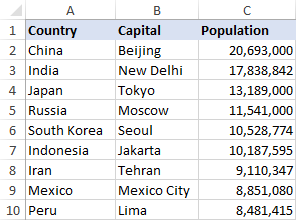To find the population of a certain capital, say the capital of Japan, use the following INDEX MATCH formula:

`=INDEX(C2:C10, MATCH("Japan", A2:A10, 0))`

Now, let's analyze what each component of this formula actually does:

• The MATCH function searches for the lookup value "Japan" in the range A2:A10, and returns the number 3, because "Japan" is third in the lookup array.
• The row number goes directly to the row_num argument of INDEX instructing it to return a value from that row.

So, the above formula turns into a simple INDEX(C2:C,3) that says to search in cells C2 through C10 and pull the value from the 3rd cell in that range, i.e. C4 because we start counting from the second row.

Don't want to hardcode the city in the formula? Input it in some cell, say F1, supply the cell reference to MATCH, and you will get a dynamic lookup formula:

`=INDEX(C2:C10, MATCH(F1,A2:A10,0))`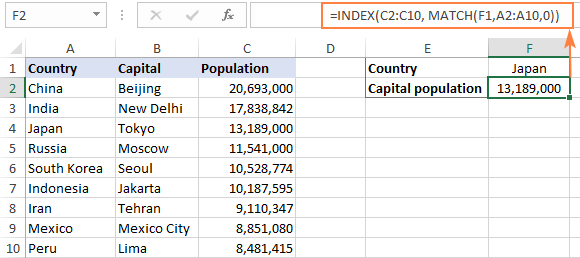Important note! The number of rows in the array argument of INDEX should match the number of rows in the lookup_array argument of MATCH, otherwise the formula will produce an incorrect result.

Wait, wait… why don't we simply use the following Vlookup formula? What's the point in wasting time trying to figure out the arcane twists of Excel MATCH INDEX?

`=VLOOKUP(F1, A2:C10, 3, FALSE)`

In this case, no point at all :) This simple example is for demonstration purposes only, so that you get a feel of how the INDEX and MATCH functions work together. Other examples that follow below will show you the real power of this combination that easily copes with many complex scenarios when VLOOKUP stumbles.

Tips:

## INDEX MATCH vs. VLOOKUP

When deciding which function to use for vertical lookups, most Excel gurus agree that INDEX MATCH is far better than VLOOKUP. However, many people still stay with VLOOKUP, firstly, because it's simpler and, secondly, because they do not fully understand all benefits of using the INDEX MATCH formula in Excel. Without such understanding no one is willing to invest their time to learn a more complex syntax.

Below, I will point out the key advantages of MATCH INDEX over VLOOKUP, and you decide whether it's a worthy addition to your Excel arsenal.

### 4 main reasons to use INDEX MATCH instead of VLOOKUP

1. Right to left lookup. As any educated user knows, VLOOKUP cannot look to its left, meaning your lookup value should always reside in the leftmost column of the table. INDEX MATCH can do left lookup with ease! The following example shows it in action: How to Vlookup a value to the left in Excel.
2. Insert or delete columns safely. VLOOKUP formulas get broken or delivers incorrect results when a new column is deleted from or added to a lookup table because VLOOKUP's syntax requires specifying the index number of the column you want to pull the data from. Naturally, when you add or delete columns, the index number changes.

With INDEX MATCH, you specify the return column range, not an index number. As the result, you are free to insert and remove as many columns as you want without worrying about updating every associated formula.

3. No limit for a lookup value's size. When using the VLOOKUP function, the total length of your lookup criteria cannot exceed 255 characters, otherwise you will end up having the #VALUE! error. So, if your dataset contains long strings, INDEX MATCH is the only working solution.
4. Higher processing speed. If your tables are relatively small, there will hardly be any significant difference in Excel performance. But if your worksheets contain hundreds or thousands of rows, and consequently hundreds or thousands of formulas, MATCH INDEX will work much faster than VLOOKUP because Excel will have to process only the lookup and return columns rather than the entire table array.

VLOOKUP's impact on Excel's performance may be especially noticeable if your workbook contains complex array formulas like VLOOKUP and SUM. The point is that checking each value in the array requires a separate call of the VLOOKUP function. So, the more values your array contains and the more array formulas you have in a workbook, the slower Excel performs.

## Excel INDEX MATCH - formula examples

Knowing the reasons to learn the MATCH INDEX function, let's get to the most interesting part and see how you can apply the theoretical knowledge in practice.

### INDEX MATCH formula to look up from right to left

As already mentioned, VLOOKUP cannot look at its left. So, unless your lookup values are is the leftmost column, there's no chance that a Vlookup formula will bring you the result you want. The INDEX MATCH function in Excel is more versatile and does not really care where the lookup and return columns are located.

For this example, we will add the Rank column to the left of our sample table and try to figure out how the Russian capital, Moscow, ranks in terms of population.

With the lookup value in G1, use the following formula to search in C2:C10 and return a corresponding value from A2:A10:

`=INDEX(A2:A10,MATCH(G1,C2:C10,0))`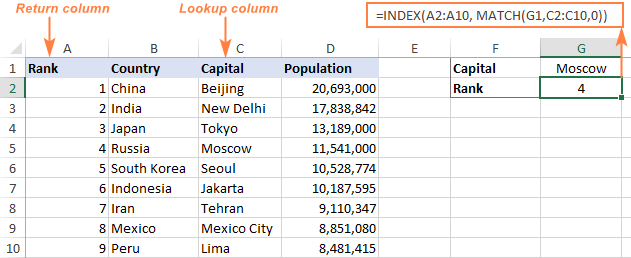Tip. If you plan to use your INDEX MATCH formula for more than one cell, be sure to lock both ranges with absolute cell references (like \$A\$2:\$A\$10 and \$C\$2:4C\$10) so they won't get distorted when copying the formula.

### INDEX MATCH MATCH to search in rows and columns

In the above examples, we used INDEX MATCH as the replacement for classic VLOOKUP to return a value from a predefined one-column range. But what if you need to look up in multiple rows and columns? In other words, what if you want to perform the so-called matrix or two-way lookup?

This may sound tricky, but the formula is very similar to the basic Excel INDEX MATCH function, with just one difference. Guess what?

Simply, use two MATCH functions – one to get a row number and the other to get a column number. And I congratulate those of you who have guessed right :)

INDEX (array, MATCH (vlookup value, column to look up against, 0), MATCH (hlookup value, row to look up against, 0))

And now, please take a look at the below table and let's build an INDEX MATCH MATCH formula to find the population (in millions) in a given country for a given year.

With the target country in G1 (vlookup value) and the target year in G2 (hlookup value), the formula takes this shape:

`=INDEX(B2:D11, MATCH(G1,A2:A11,0), MATCH(G2,B1:D1,0))`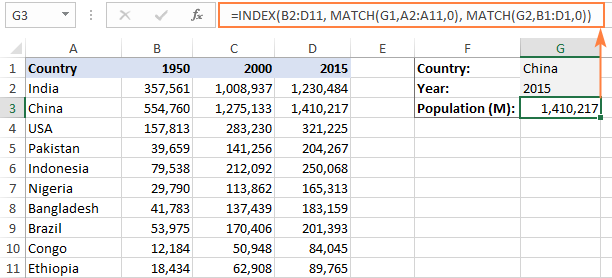#### How this formula works

Whenever you need to understand a complex Excel formula, break it down into smaller parts and see what each individual function does:

`MATCH(G1,A2:A11,0)` – searches through A2:A11 for the value in cell G1 ("China") and returns its position, which is 2.

`MATCH(G2,B1:D1,0))` – searches through B1:D1 to get the position of the value in cell G2 ("2015"), which is 3.

The above row and column numbers go to the corresponding arguments of the INDEX function:

`INDEX(B2:D11, 2, 3)`

As the result, you get a value at the intersection of the 2nd row and 3rd column in the range B2:D11, which is the value in cell D3. Easy? Yep!

### Excel INDEX MATCH to look up multiple criteria

If you had a chance to read our Excel VLOOKUP tutorial, you have probably already tested a formula to Vlookup with multiple criteria. However, a significant limitation of that approach is the necessity to add a helper column. The good news is that Excel's INDEX MATCH function can look up with two or more criteria too, without modifying or restructuring your source data!

Here's the generic INDEX MATCH formula with multiple criteria:

{=INDEX(return_range, MATCH(1, (criteria1=range1) * (criteria2=range2), 0))}

Note. This is an array formula that must be completed with the Ctrl + Shift + Enter shortcut.

In the sample table below, supposing you want to find the amount based on 2 criteria, Customer and Product.

The following INDEX MATCH formula works a treat:

`=INDEX(C2:C10, MATCH(1, (F1=A2:A10) * (F2=B2:B10), 0))`

Where C2:C10 is the range to return a value from, F1 is criteria1, A2:A10 is the range to compare against criteria1, F2 is criteria 2, and B2:B10 is the range to compare against criteria2.

Remember to enter the formula correctly by pressing Ctrl + Shift + Enter, and Excel will automatically enclose it curly brackets as shown in the screenshot: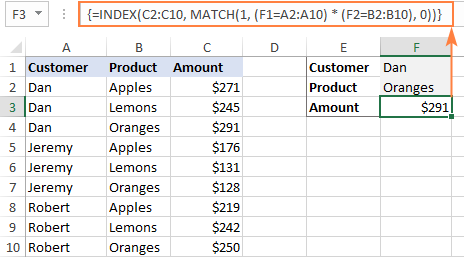If you'd rather not use array formulas in your worksheets, add one more INDEX function to the formula and complete it with a usual Enter hit: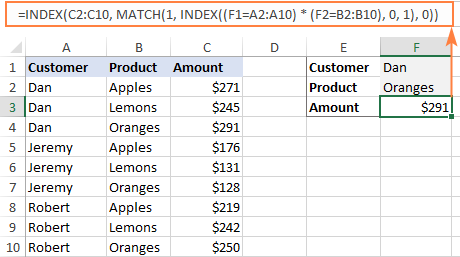#### How these formulas work

The formulas use the same approach as the basic INDEX MATCH function that looks through a single column. To evaluate multiple criteria, you create two or more arrays of TRUE and FALSE values that represent matches and non-matches for each individual criterion, and then multiply the corresponding elements of these arrays. The multiplication operation converts TRUE and FALSE into 1 and 0, respectively, and produces an array where 1's correspond to rows that match all the criteria. The MATCH function with a lookup value of 1 finds the first "1" in the array and passes its position to INDEX, which returns a value in this row from the specified column.

The non-array formula relies on the ability of the INDEX function to handle arrays natively. The second INDEX is configured with 0 row_num so that it will pass the entire column array to MATCH.

That is a high-level explanation of the formula's logic. For full details, please see Excel INDEX MATCH with multiple criteria.

### Excel INDEX MATCH with AVERAGE, MAX, MIN

Microsoft Excel has special functions to find a minimum, maximum and average value in a range. But what if you need to get a value from another cell that is associated with those values? In this case, use the MAX, MIN or AVERAGE function together with INDEX MATCH.

#### INDEX MATCH with MAX

To find the largest value in column D and return a value from column C in the same row, use this formula:

`=INDEX(C2:C10, MATCH(MAX(D2:D10), D2:D10, 0))`

#### INDEX MATCH with MIN

To locate the smallest value in column D and pull an associated value from column C, use this one:

`=INDEX(C2:C10, MATCH(MIN(D2:D10), D2:D10, 0))`

#### INDEX MATCH with AVERAGE

To work out the value closest to the average in D2:D10 and get a corresponding value from column C, this is the formula to use:

`=INDEX(C2:C10, MATCH(AVERAGE(D2:D10), D2:D10, -1 ))`

Depending on how your data is organized, supply either 1 or -1 to the third argument (match_type) of the MATCH function:

• If your lookup column (column D in our case) is sorted ascending, put 1. The formula will calculate the largest value that is less than or equal to the average value.
• If your lookup column is sorted descending, enter -1. The formula will compute the smallest value that is greater than or equal to the average value.
• If your lookup array contains a value exactly equal to the average, you can enter 0 for exact match. No sorting is required.

In our example, the populations in column D are sorted in descending order, so we use -1 for match type. As the result, we get "Tokyo" since its population (13,189,000) is the closest match that is greater than average (12,269,006).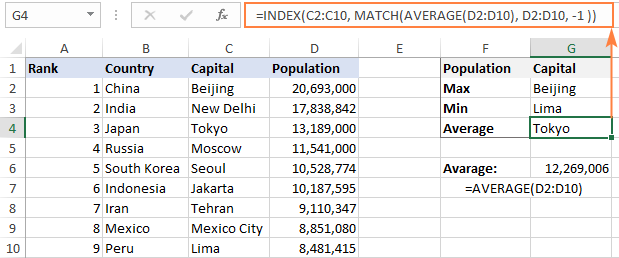You may be curious to know that VLOOKUP can perform such calculations too, but as an array formula: VLOOKUP with AVERAGE, MAX, MIN.

## Using INDEX MATCH with IFNA / IFERROR

As you have probably noticed, if an INDEX MATCH formula in Excel cannot find a lookup value, it produces an #N/A error. If you wish to replace the standard error notation with something more meaningful, wrap your INDEX MATCH formula in the IFNA function. For example:

`=IFNA(INDEX(C2:C10, MATCH(F1,A2:A10,0)), "No match is found")`

And now, if someone inputs a lookup table that does not exist in the lookup range, the formula will explicitly inform the user that no match is found: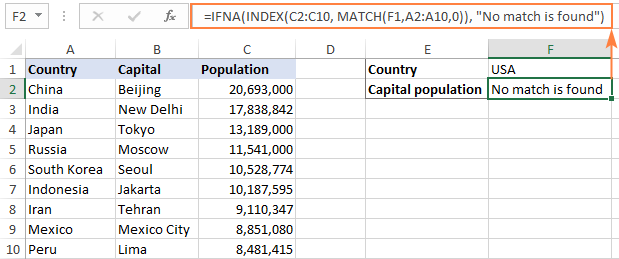If you'd like to catch all errors, not only #N/A, use the IFERROR function instead of IFNA:

`=IFERROR(INDEX(C2:C10, MATCH(F1,A2:A10,0)), "Oops, something went wrong!")`

Please keep in mind that in many situations it might be unwise to disguise all errors because they alert you about possible faults in your formula.

That's how to use INDEX and MATCH in Excel. I hope our formula examples will prove helpful for you and look forward to seeing you on our blog next week!